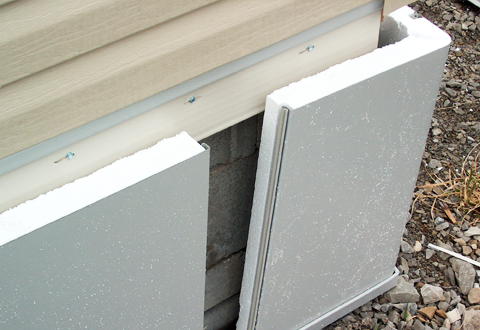## Insulated Vinyl Skirting Panel

• \$14200

Designed to individually lock each panel together for extra strength and secure fit.

Panels are adhered to 2" thick EPS Foam providing R-9 Insulating Value

Each Panel is 47" Wide x 12' Long

Interlocking panels and impact resistant

4' panels are easily installed and have fewer seams

No bracing or framing required

Corner Pieces and Trim Kit sold separately

To determine how many panels you need, make the following calculations:

Measure the lineal feet of the area to be skirted.

Example  16x80 home = 184' lineal feet (16+16+76+76)

Multiply the lineal feet x 12 = Total lineal inches.

Ex. 184' x 12 = 2,208 total lineal inches

Divide the total lineal inches / 47" panel width = Number of panels sections needed.

Ex.  2,208/47 = 47 panel sections

Measure you home from the ground to the base of the house, at each corner and halfway down each of the longest sides.  You should have 6 measurements.  Add them together and divide by 6 = Average height of the home off the ground.

Ex. 26" + 28" + 35" + 37" + 42" + 40" = 208"

208"/6 = 34.67" average height

Divide 144"(total height of panel in inches) / Average Height = Number of cuts that can be made in each panel.

Ex. 144" / 34.67" = 4.15 cuts out of each panel.

Divide the number of total panels sections needed / number of cuts per panel = Number of 12' panels needed

Ex. 47 / 4.15 = 11.3 total panels needed to skirt the home

This a close approximate figure.  You may need to buy an additional panel or two to make sure you have enough material to complete your job.

More Products in this Category